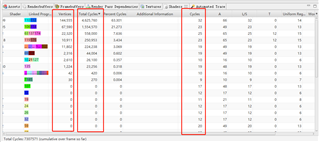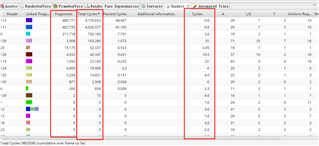# Question about fragment count statistic in graphics analyzer1.We want to know whether the number of Vertices and Fragments counted here is the number after culling (HiZ, geometry culling, earlyz, fpk...)?

2.We found that the total cost of each shader = Cycles*Fragments or Cycles*Vertices, which parameter is the accurate GPU consumption data?

3.Does the Cycles data come from the estimation of Mali Offline Compiler? Then multiply the number directly to get the total cycles overhead?

4.In general, can we analyze and locate the cost of a specific drawcall by using Fragment Count Statistic in Graphics Analyzer? Is it real GPU overhead data?

• Hi Shawn,

1.We want to know whether the number of Vertices and Fragments counted here is the number after culling (HiZ, geometry culling, earlyz, fpk...)?

Vertex count is the number of unique indices seen when the draw call was made. Fragment count is based on an estimate given screenshot capture, which includes hier-z, and early-zs, but doesn't handle FPK hidden surface removal.

2.We found that the total cost of each shader = Cycles*Fragments or Cycles*Vertices, which parameter is the accurate GPU consumption data?

Both use processing cycles, so you need both costs.

3.Does the Cycles data come from the estimation of Mali Offline Compiler? Then multiply the number directly to get the total cycles overhead?

Yes, the shader costing is from the Mali Offline Compiler for a Mali-T880 GPU. The number user is the average of the shortest and longest path, so may not reflect exactly what happens based on runtime state.

4.In general, can we analyze and locate the cost of a specific drawcall by using Fragment Count Statistic in Graphics Analyzer? Is it real GPU overhead data?

No, the tool doesn't give a means to cost a specific draw call, only programs.

The cost is entirely estimated in software it's not a direct measure from the hardware.

HTH,
Pete

More questions in this forum

3395 views
3 replies
Latest

800 views
Latest

739 views